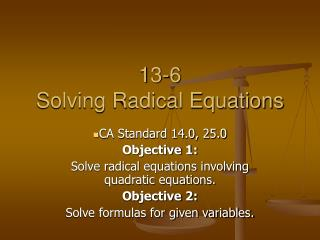DownloadDownload PresentationTélécharger la présentation- - - - - - - - - - - - - - - - - - - - - - - - - - - E N D - - - - - - - - - - - - - - - - - - - - - - - - - - -
##### Presentation Transcript

1. 13-6Solving Radical Equations CA Standard 14.0, 25.0 Objective 1: Solve radical equations involving quadratic equations. Objective 2: Solve formulas for given variables.

2. Standards 14.0, 25.0 • Using factoring to solve quadratic equations; use properties of the number system to justify each step of a procedure.

3. #1 Solve Standard Form

4. Check

5. #2 Solve Standard Form

6. Check

7. #3 Solution Standard Form

8. Check

9. Solve the following equations

10. #4 Solution Standard Form

11. Check

12. #5 Solution Standard Form

13. Check

14. #6 Solve for the indicated variable

15. Solve the following equations

16. #7 Solve for the indicated variable

17. #8 Solve for the indicated variable